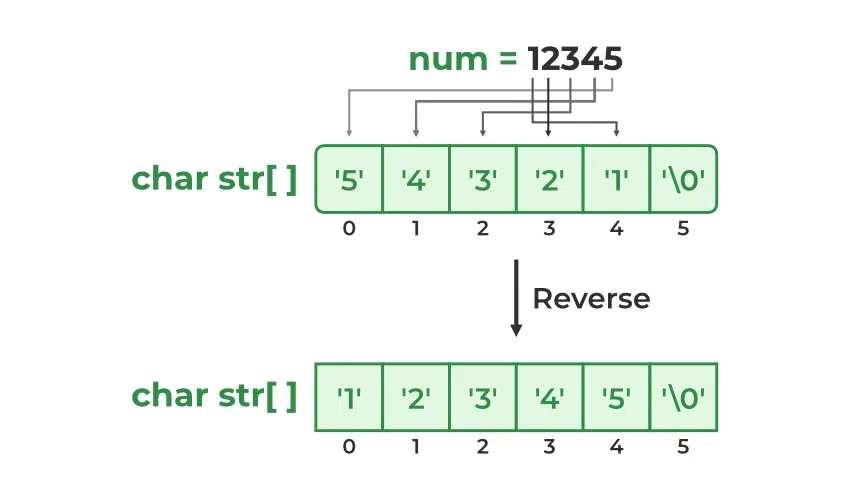GFG App
Open AppBrowser
Continue

The itoa function converts an integer into a null-terminated string. It can convert negative numbers too. It is a standard library function that is defined inside stdlib.h header file.

### Syntax of itoa in C

`char* itoa(int num, char* buffer, int base);`

In this article, we create a C Program to implement a custom function that will be able to convert the given integer to a null-terminated string similar to itoa() function in C.

## Basic Principle

Individual digits of the given number must be processed and their corresponding characters must be put in the given string. Using repeated division by the given base, we get individual digits from the least significant to the most significant digit. But in the output, these digits are needed in reverse order. Therefore, we reverse the string obtained after repeated division and return it.

## Algorithm

The working algorithm for the given approach is as follows:

• STEP 1: The number is passed to the function along with its base and destination string pointer as the arguments.
• STEP 2A: If the number is zero, then return the string: “0\0”.
• STEP 2B: If the number is not zero, then
• STEP 3: Set the isNegative flag to true if the number is negative.
• STEP 4: We get the least significant digit by evaluating the remainder of the number divided by the base.
• STEP 5A: If the remainder is greater than 9 (i.e. base is higher than 10), then put the corresponding alphabetical character in the string.
• STEP 5B: If the reminder is less than or equal to 9, put the corresponding numeric character in the string.
• STEP 6: Decrease the number of digits by one by dividing the number by the base.
• STEP 7: Repeat the steps from 4 to 6 till the number is less than or equal to zero.
• STEP 8: Now, if the isNegative flag is true, then put a ( ) negative sign at the end.
• STEP 9: Put ‘\0’ at the end of the string.
• STEP 10: After that, reverse the string as the numbers in the string will be in reverse as compared to the actual integer.
• STEP 11: Return the string address.Working of Custom itoa()

## C

 `// C program to implement itoa()` `#include ` `#include `   `// A utility function to reverse a string` `void` `reverse(``char` `str[], ``int` `length)` `{` `    ``int` `start = 0;` `    ``int` `end = length - 1;` `    ``while` `(start < end) {` `        ``char` `temp = str[start];` `        ``str[start] = str[end];` `        ``str[end] = temp;` `        ``end--;` `        ``start++;` `    ``}` `}` `// Implementation of citoa()` `char``* citoa(``int` `num, ``char``* str, ``int` `base)` `{` `    ``int` `i = 0;` `    ``bool` `isNegative = ``false``;`   `    ``/* Handle 0 explicitly, otherwise empty string is` `     ``* printed for 0 */` `    ``if` `(num == 0) {` `        ``str[i++] = ``'0'``;` `        ``str[i] = ``'\0'``;` `        ``return` `str;` `    ``}`   `    ``// In standard itoa(), negative numbers are handled` `    ``// only with base 10. Otherwise numbers are` `    ``// considered unsigned.` `    ``if` `(num < 0 && base == 10) {` `        ``isNegative = ``true``;` `        ``num = -num;` `    ``}`   `    ``// Process individual digits` `    ``while` `(num != 0) {` `        ``int` `rem = num % base;` `        ``str[i++] = (rem > 9) ? (rem - 10) + ``'a'` `: rem + ``'0'``;` `        ``num = num / base;` `    ``}`   `    ``// If number is negative, append '-'` `    ``if` `(isNegative)` `        ``str[i++] = ``'-'``;`   `    ``str[i] = ``'\0'``; ``// Append string terminator`   `    ``// Reverse the string` `    ``reverse(str, i);`   `    ``return` `str;` `}`   `// Driver program to test implementation of itoa()` `int` `main()` `{` `    ``char` `str;` `    ``printf``(``"Number: %d\nBase: %d\tConverted String: %s\n"``,` `           ``1567, 10, citoa(1567, str, 10));` `    ``printf``(``"Base: %d\t\tConverted String: %s\n"``, 2,` `           ``citoa(1567, str, 2));` `    ``printf``(``"Base: %d\t\tConverted String: %s\n"``, 8,` `           ``citoa(1567, str, 8));` `    ``printf``(``"Base: %d\tConverted String: %s\n"``, 16,` `           ``citoa(1567, str, 16));` `    ``return` `0;` `}`

Output

```Number: 1567
Base: 10    Converted String: 1567
Base: 2        Converted String: 11000011111
Base: 8        Converted String: 3037
Base: 16    Converted String: 61f```

Time Complexity: O(logb num),

Auxiliary Space: O(1)